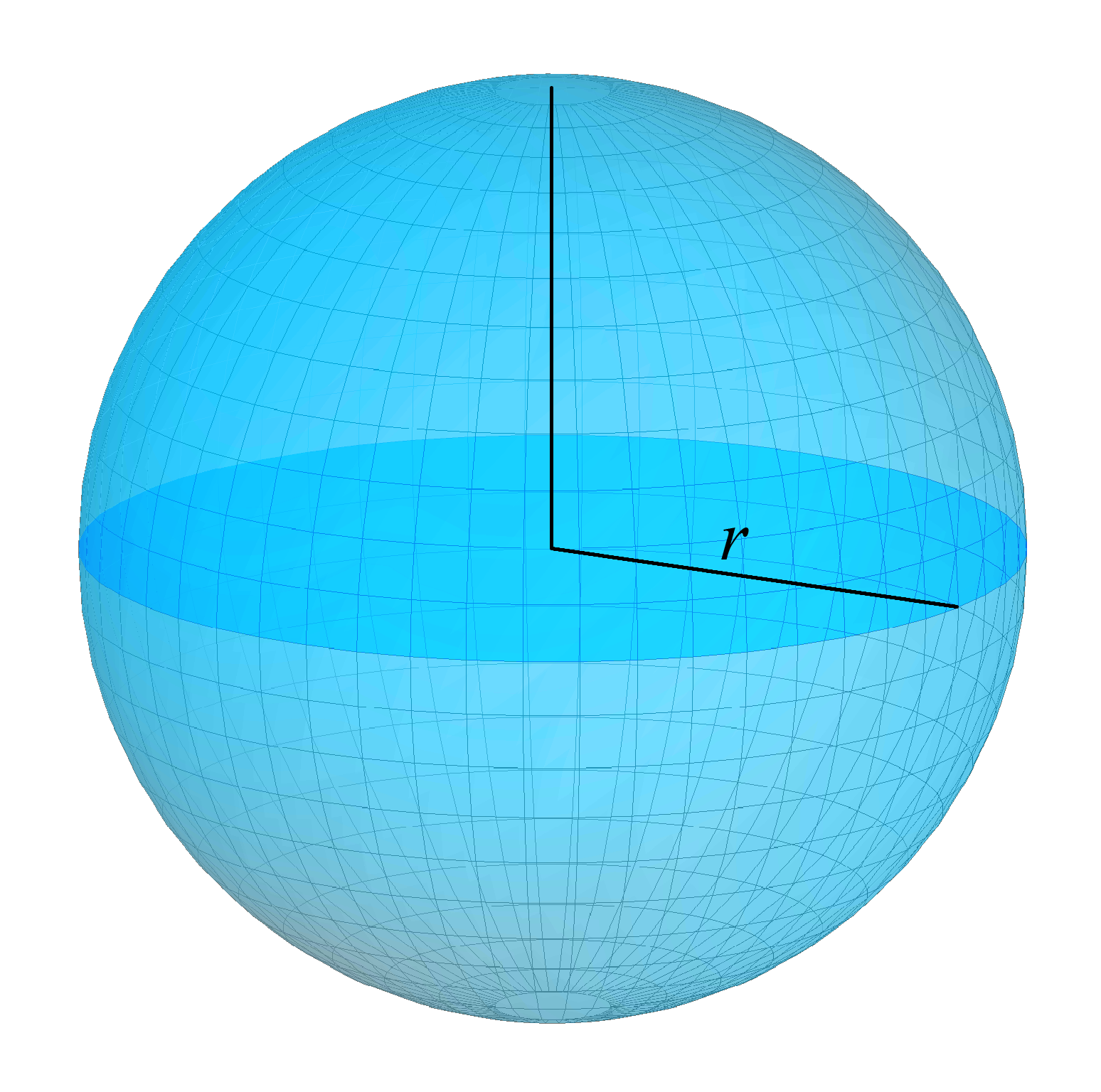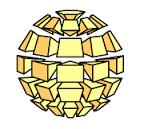# Area & Volume Of Sphere

Area of sphere or total surface area of sphere is the region covered by a surface of a spherical object in a three-dimensional space. Since the sphere is a complete curved shape therefore the curved surface area is equal to the total area of sphere. It is also called lateral surface area.

 Surface Area of Sphere = 4πr², where r is the radius of sphere.

A sphere is a solid figure bounded by a curved surface such that every point on the surface is the same distance from the centre.  In other words, a sphere is a perfectly round geometrical object in three-dimensional space, just like a surface of a round ball. The distance from the center to the outer surface of sphere is called its radius. The surface area of a sphere is defined as the amount of region covered by the surface of a sphere and is equal to 4πr²The volume of sphere is the space occupied by it in 3d space.

## What is the Sphere?

A sphere is mathematically defined as the set of points that are all at the same distance from a given point but in three-dimensional space.

The distance from the centre to the outermost surface is called the radius of the sphere and the line that connects two points on the sphere and is twice the length of the radius is called diameter.Area & Volume Of Sphere

### Sphere Properties

Some of the important properties of sphere are as follows:

• Sphere has no face and edge
• A sphere is perfectly symmetrical
• It is not a polyhedron
• All the points on the surface are equidistant from the centre of the sphere.
• It has only one surface (but not the face)

## Surface Area of Sphere

A sphere is the 3- D shape where the curved surface area equals to the total surface area of the figure. The curved surface area is the area in which only the area of the curved part is covered. The formula does not take into account the circular base.

The total surface area, on the other hand, is a combination of the curved area along with the area of the base The formula for the total surface area  and the curved surface area of the sphere is given below

Surface area (TSA) = CSA = 4πr² square units

### How to Find Area of a Sphere?

To find the area of the sphere firstly, follow the below steps:

• Find the radius of the sphere
• Mention the value of radius in the surface area formula, i.e. (4πr²)
• Solve the expression and evaluate the final value

## Area of Hemisphere

Hemisphere is also a 3d shape, which is just half of the sphere. When a plane cuts the sphere in two equal halves, we get a hemisphere.

The total surface area (TSA) of hemisphere is equal to the sum of its curved surface area and base area (circular base).

Therefore,

TSA (hemisphere) = Half of area of sphere + Base area

TSA = 2πr² + πr²

TSA = 3πr²

## Volume of Sphere

The volume of the sphere is defined as the number of cubic units needed to fill a sphere.

The formula for volume of sphere is given by:

 Volume = 4/3 πr3 (Cubic Units)

Unit: S.I unit is given cubic meters ( m³)

The derivation is given as:V=Volume of the Sphere

= Sum of the volumes of all pyramids

= 1/3A1r + 1/3A2r  + 1/3A3r+….+1/3Anr

= 1/3(Surface area of the sphere) r

=1/3(4πr2)×r

=4/3(πr3)

In the above derivation, the sphere in Fig:2 is divided into pyramids, and the volume of the sphere is equal to the volume of all the pyramids as shown in the figure. The total volume is calculated by the summation of the pyramids volumes.

### How to Find Volume of Sphere?

Steps for Finding the Volume of a Sphere:

Step 1: Find the radius of a sphere and cubic it.

Step 2: Take the product of 4/3 π and the cube of the radius of the sphere.

Step 3: Write the answer in the proper cubic unit of measurement.

## Solved Examples

Example 1:

Find the volume of a sphere of diameter 12 m, rounding your answer to two decimal places(using pi = 3.14).

Solution:

Diameter of the sphere (d) = 12 m

Step 1:

Find the radius of the sphere:

Radius of the sphere (r) = d/2 = 12/2 = 6 m

Step 2:

Volume of the sphere (V) = 4/3 π r3

V = 4/3 x 3.14 x 63

V = 4/3 x 3.14 x 6 x 6 x 6

V = 904.32

Hence the volume of the sphere is 904.32 m3 .

Example 2:

A plane passes through the centre of a sphere and forms a circle with a radius of 12 feet. What is the surface area of the sphere?

Solution:

Radius of the sphere = 12 feet

Volume of the sphere (V) = 4 π r2

= 4 x 3.14 x 12 x 12

= 1808.64

Hence area of the sphere is 1808.64 feet2.

Example 3:

A spherical ball has a surface area of 2464 sq. cm. Find the radius of the ball, correct to 2 decimal places(using pi = 22/7)

Solution:

Step 1:

Surface area of a sphere = 2464 cm2

To find: Radius of a sphere

Step 2:

Surface area a sphere (SA) = 4 π r2

Radius of a sphere (r) = $$\sqrt{\frac{Surface \ Area}{4\pi}}$$

r = $$\sqrt{\frac{2464 \times 7}{4 \times 22}}$$

= 14

Hence the radius of a sphere is 14 cm.

## Practice Questions

1. Find the area of sphere if the radius is equal to 13cm.
2. Find the radius of sphere if the surface area is equal to 400 sq.cm.
3. Find the area of sphere if the diameter of sphere is 30 cm.
4. Find the area of sphere if the volume of sphere is 330 cubic centimeters.

For more Maths-related articles, register with BYJU’S – The Learning App and download the app today to learn with ease.

## Frequently Asked Questions – FAQs

### What is the area of sphere?

Surface area of sphere is the region occupied by its surface in the three-dimensional space.

### What is the formula for area of sphere?

The formula to find the surface area of sphere is 4 times of pi (π) and radius-squared (r2).
Surface area of sphere = 4 π r2

### What is the volume of sphere?

The volume of sphere is the space occupied by it in three dimensional space. The formula to find the volume is 4/3 π r3.